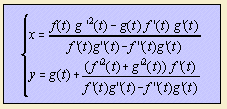# curvature curve

## main

There are four derived curves that are related to curvature:

Given a curve C1, the evolute is the curve C2 defined by the loci of the centers of curvature of C1. In other words: construct in each point P of curve C1 a circle that is a tangent to C1 in P; then the center of the circle belongs to C2.

When C1 is given by (x, y) = (f(t), g(t)), then C2 has the form:If a line l rolls without slipping as a tangent along a curve C1, then the path of a point P on l forms a new curve C2, the involute of C1. Involution is the reverse operation of evolution: if C2 is the involute of C1, then C1 is the evolute of C2.
You might ask yourself whether there exists a curve whose involute is exactly the same curve. Well, there are two curves with this property:

Besides, there are some curves whose involute is the same curve, but not equal in position or magnitude:

Some other involute-evolute couples are:

 involute evolute ellipse astroid epicycloid epicycloid (similar, but smaller) involute of a circle circle orthotomic caustic tractrix catenary roulette: when a line is rolling over curve C, the path of any point on the line. any curve C

In fact, the evolute of a curve is the same as the envelope of its normal.

The radial is a variation on the evolute: draw, from a fixed point, lines parallel to the radii of curvature, with the same length as the radii. The set of end points is the radial. The logarithmic spiral is the curve whose radial is the curve itself.

Radials of some other curves are:

 base curve radial astroid quadrifolium catenary kampyle of Eudoxus Cayley's sextic nephroid cycloid circle deltoid trifolium epicycloid rhodonea parabola semi cubical parabola tractrix kappa curve

Given a curve, the curvature κ is defined as the inclination per arc length: κ(s) = dφ/ds.

This curvature can be expressed for a curve y = f (x) as follows:If the curvature is positive (>= 0), we speak of a convex curve.
If the curvature is strictly positive (>0),  we speak of a strictly convex curve.

If the curvature is negative (<= 0), we speak of a concave curve.
If the curvature is strictly negative (<0),  we speak of a strictly concave curve.

Given the curvature as function of the arc length, you can look for the representing curve.
Some example curves are the following:

 κ = dφ/ds curve 1 circle 1 / s logarithmic spiral 1 / √s involute of a circle s Euler´s spiral s2 double clothoid ∑ai si polynomial spiral a sin s meander curve

The radius of curvature R is the reciprocal of the absolute value of the curvature κ, so that R = 1/κ.
The Cesaró equation writes a curve in terms of a radius of curvature R and an arc length s.